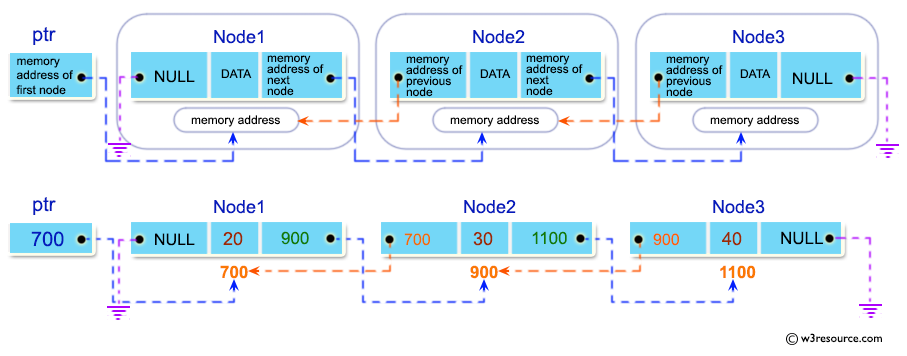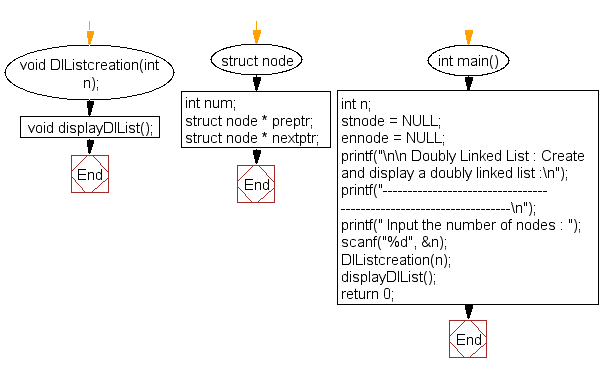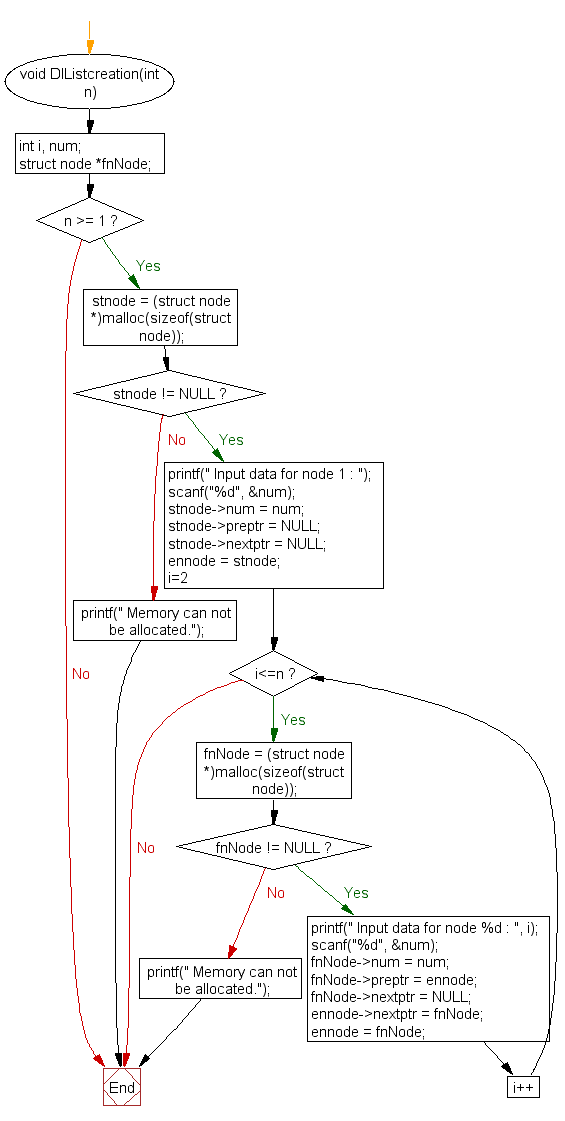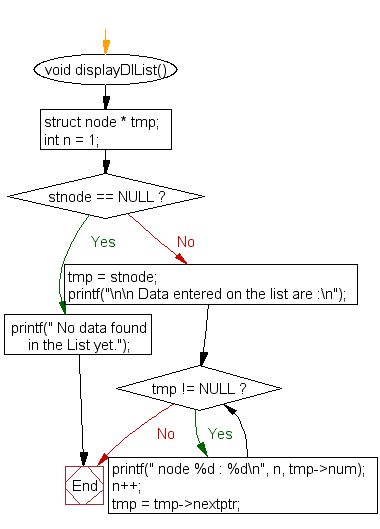﻿ C Program: Create and display a doubly linked list - w3resource

# C Exercises: Create and display a doubly linked list

## C Doubly Linked List : Exercise-1 with Solution

Write a program in C to create and display a doubly linked list.

Pictorial Presentation:Sample Solution:

C Code:

``````#include <stdio.h>
#include <stdlib.h>

struct node {
int num;
struct node * preptr;
struct node * nextptr;
}*stnode, *ennode;

void DlListcreation(int n);
void displayDlList();

int main()
{
int n;
stnode = NULL;
ennode = NULL;
printf("\n\n Doubly Linked List : Create and display a doubly linked list :\n");
printf("-------------------------------------------------------------------\n");

printf(" Input the number of nodes : ");
scanf("%d", &n);

DlListcreation(n);
displayDlList();
return 0;
}

void DlListcreation(int n)
{
int i, num;
struct node *fnNode;

if(n >= 1)
{
stnode = (struct node *)malloc(sizeof(struct node));

if(stnode != NULL)
{
printf(" Input data for node 1 : "); // assigning data in the first node
scanf("%d", &num);

stnode->num = num;
stnode->preptr = NULL;
stnode->nextptr = NULL;
ennode = stnode;
// putting data for rest of the nodes
for(i=2; i<=n; i++)
{
fnNode = (struct node *)malloc(sizeof(struct node));
if(fnNode != NULL)
{
printf(" Input data for node %d : ", i);
scanf("%d", &num);
fnNode->num = num;
fnNode->preptr = ennode;    // new node is linking with the previous node
fnNode->nextptr = NULL;

ennode->nextptr = fnNode;   // previous node is linking with the new node
ennode = fnNode;            // assign new node as last node
}
else
{
printf(" Memory can not be allocated.");
break;
}
}
}
else
{
printf(" Memory can not be allocated.");
}
}
}
void displayDlList()
{
struct node * tmp;
int n = 1;
if(stnode == NULL)
{
printf(" No data found in the List yet.");
}
else
{
tmp = stnode;
printf("\n\n Data entered on the list are :\n");

while(tmp != NULL)
{
printf(" node %d : %d\n", n, tmp->num);
n++;
tmp = tmp->nextptr; // current pointer moves to the next node
}
}
}
```
```

Sample Output:

``` Doubly Linked List : Create and display a doubly linked list :
-------------------------------------------------------------------
Input the number of nodes : 3
Input data for node 1 : 2
Input data for node 2 : 5
Input data for node 3 : 8

Data entered on the list are :
node 1 : 2
node 2 : 5
node 3 : 8
```

Flowchart:DlListcreation() :displayDlList() :C Programming Code Editor:

What is the difficulty level of this exercise?

Test your Programming skills with w3resource's quiz.

﻿